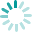## Stacked triangles

https://www.knittingunlimited.com/2022/06/blanket-29-stacked-triangles.html

Shown with a cast-on count of 47 stitches.• Row 1 (RS): [K1, p1] twice, k1, p6, [k1, p11] twice, k1, p6, [k1, p1] twice, k1.
• Row 2: [K1, p1] twice, k7, p1, [k11, p1] twice, k7, [p1, k1] twice.
• Row 3: [K1, p1] twice, k1, p5, [k3, p9] twice, k3, p5, [k1, p1] twice, k1.
• Row 4: [K1, p1] twice, k6, p3, [k9, p3] twice, k6, [p1, k1] twice.
• Row 5: [K1, p1] twice, k1, p4, [k5, p7] twice, k5, p4, [k1, p1] twice, k1.
• Row 6: [K1, p1] twice, k5, p5, [k7, p5] twice, k5, [p1, k1] twice.
• Row 7: [K1, p1] twice, k1, p3, [k7, p5] twice, k7, p3, [k1, p1] twice, k1.
• Row 8: [K1, p1] twice, k4, p7, [k5, p7] twice, k4, [p1, k1] twice.
• Row 9: [K1, p1] twice, k1, p2, [k9, p3] twice, k9, p2, [k1, p1] twice, k1.
• Row 10: [K1, p1] twice, k3, p9, [k3, p9] twice, k3, [p1, k1] twice.
• Row 11: [K1, p1] 3 times, [k11, p1] twice, k11, [p1, k1] 3 times.
• Row 12: [K1, p1] twice, k2, p11, [k1, p11] twice, k2, [p1, k1] twice.
• Row 13: [K1, p1] twice, k2, [p11, k1] twice, p11, k2, [p1, k1] twice.
• Row 14: [K1, p1] 3 times, k11, [p1, k11] twice, [p1, k1] 3 times.
• Row 15: [K1, p1] twice, k3, [p9, k3] twice, p9, k3, [p1, k1] twice.
• Row 16: [K1, p1] twice, k1, p2, k9, [p3, k9] twice, p2, [k1, p1] twice, k1.
• Row 17: [K1, p1] twice, k4, [p7, k5] twice, p7, k4, [p1, k1] twice.
• Row 18: [K1, p1] twice, k1, p3, k7, [p5, k7] twice, p3, [k1, p1] twice, k1.
• Row 19: [K1, p1] twice, k5, [p5, k7] twice, p5, k5, [p1, k1] twice.
• Row 20: [K1, p1] twice, k1, p4, k5, [p7, k5] twice, p4, [k1, p1] twice, k1.
• Row 21: [K1, p1] twice, k6, [p3, k9] twice, p3, k6, [p1, k1] twice.
• Row 22: [K1, p1] twice, k1, p5, k3, [p9, k3] twice, p5, [k1, p1] twice, k1.
• Row 23: [K1, p1] twice, k7, [p1, k11] twice, p1, k7, [p1, k1] twice.
• Row 24: [K1, p1] twice, k1, p6, k1, [p11, k1] twice, p6, [k1, p1] twice, k1.